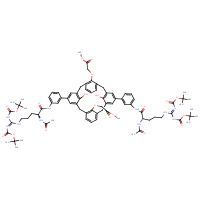Structure InformationCompound Identification

SMILES

COC(=O)COC1=C2CC3=CC(=CC(CC4=C(OCC(=O)OC)C(CC5=C(O)C(CC1=CC=C2)=CC(=C5)C1=CC(NC(=O)[C@H](CCCN\C(NC(=O)OC(C)(C)C)=N/C(=O)OC(C)(C)C)NC(C)=O)=CC=C1)=CC=C4)=C3O)C1=CC(NC(=O)[C@H](CCCN\C(NC(=O)OC(C)(C)C)=N/C(=O)OC(C)(C)C)NC(C)=O)=CC=C1

InChIKey

InChIKey=UBBDSGSJPBNURU-VKOZLBNWSA-N

Formula

C82H102N10O20

Mass

1547.768

Export to:

Taxonomic Classification

Taxonomy Tree

Kingdom

Organic compounds

Superclass

Organic acids and derivatives

Class

Carboxylic acids and derivatives

Subclass

Amino acids, peptides, and analogues

Intermediate Tree Nodes

Amino acids and derivatives - Alpha amino acids and derivatives

Direct Parent

Arginine and derivatives

Alternative Parents

Molecular Framework

Aromatic homopolycyclic compounds

Substituents

Arginine or derivatives - N-acyl-alpha amino acid or derivatives - Alpha-amino acid amide - Phenoxyacetate - Anilide - Phenol ether - N-arylamide - Alkyl aryl ether - Phenol - Monocyclic benzene moiety - Fatty acyl - Fatty amide - Benzenoid - Dicarboxylic acid or derivatives - Carbamic acid ester - Methyl ester - Acetamide - Secondary carboxylic acid amide - Carboxamide group - Carboxylic acid ester - Guanidine - Organic 1,3-dipolar compound - Propargyl-type 1,3-dipolar organic compound - Ether - Carboximidamide - Organic oxygen compound - Organic nitrogen compound - Hydrocarbon derivative - Carbonyl group - Organic oxide - Organooxygen compound - Imine - Organonitrogen compound - Aromatic homopolycyclic compound

Description

This compound belongs to the class of organic compounds known as arginine and derivatives. These are compounds containing arginine or a derivative thereof resulting from reaction of arginine at the amino group or the carboxy group, or from the replacement of any hydrogen of glycine by a heteroatom.

External Descriptors

Not available